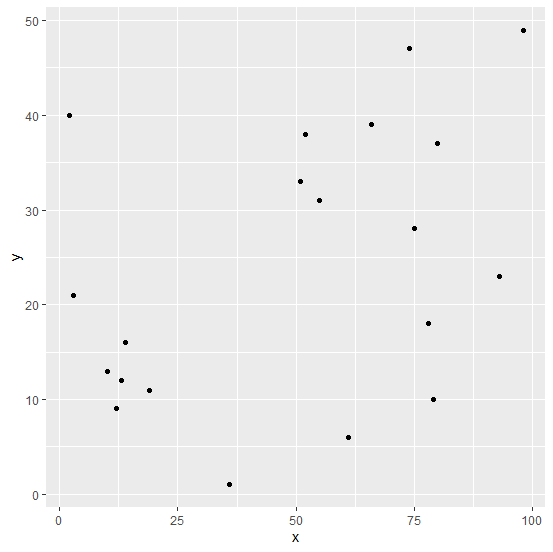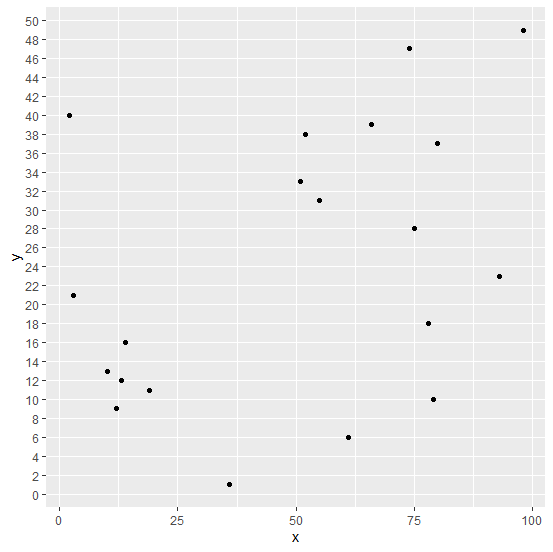# How to change the gridlines of Y-axis on a chart created by using ggplot2 in R?

Normally, the gridlines on a plot created by using ggplot2 package are a little far from each other but sometimes the plot looks better if the gridlines are close to each other, therefore, we might want to do so. This can be done by setting the minor_breaks and breaks using scale_y_continuous if the Y-axis plots a continuous variable.

## Example

Consider the below data frame −

Live Demo

> x<-sample(1:100,20)
> y<-sample(1:50,20)
> df<-data.frame(x,y)
> df

## Output

   x  y
1 14 16
2 36 1
3 78 18
4 61 6
5 19 11
6 2 40
7 93 23
8 10 13
9 3 21
10 55 31
11 75 28
12 51 33
13 13 12
14 80 37
15 98 49
16 66 39
17 79 10
18 52 38
19 74 47
20 12 9

Loading ggplot2 package and creating a scatterplot between x and y −

> library(ggplot2)
> ggplot(df,aes(x,y))+geom_point()

## OutputChanging the gridlines in the plot −

> ggplot(df,aes(x,y))+geom_point()+scale_y_continuous(minor_breaks=seq(0,50,2),breaks
=seq(0,50,2))

## Output